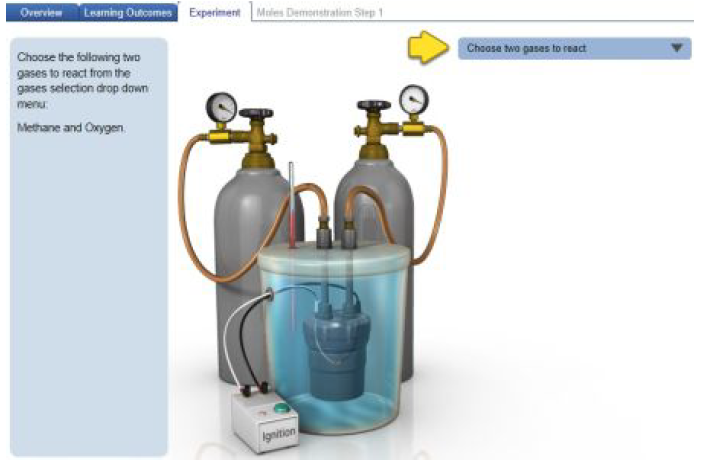# Problem: When solving problems involving stoichiometric coefficients, the first step is to make sure you have a balanced chemical equation. Then, you determine the limiting reagent by using the coefficients from the balanced equation. You can keep track of the amounts of all reactants and products before and after a reaction using an ICF table (as shown in the Simulation). Completing the ICF table will also allow you to determine the limiting reagent, and the amount of product formed is based on assuming that the reaction runs to completion with 100% yield. Parts A and C explore these steps in more detail. Let us consider another reaction.Ammonia and oxygen react to form nitrogen monoxide and water. Construct your own balanced equation to determine the amount of NO and H2O that would form when 2.86 mol NH3 and 4.95 mol O2 react.Express the amounts in moles to two decimal places separated by a comma.Click on the image below to explore this simulation, which allows you run reactions between various gases and specify their amounts. When you click the simulation link, you will be able toselect among Overview, Learning Outcomes, and Experiment tabs.When the simulation is opened, you should see an overview describing how stoichiometry relates to the changes in the amounts of reactants and products in a reaction. Clicking the menu tabs toward the top of the screen can switch among an overview of stoichiometry, the learning outcomes of the simulation, and three experiments. Two of the experiments are guided tutorials that demonstrate how moles (Run Moles Demonstration) and both mass and moles (Run Grams Demonstration) change during a reaction. You can also run your own experiment by clicking Run Experiment. For any of the experiments, follow the step-by-step procedures that are explained on the left, and your interactions within the guided tutorial will be guided by an undulating yellow arrow.

###### FREE Expert Solution
82% (38 ratings)View Complete Written Solution
###### Problem Details

When solving problems involving stoichiometric coefficients, the first step is to make sure you have a balanced chemical equation. Then, you determine the limiting reagent by using the coefficients from the balanced equation. You can keep track of the amounts of all reactants and products before and after a reaction using an ICF table (as shown in the Simulation). Completing the ICF table will also allow you to determine the limiting reagent, and the amount of product formed is based on assuming that the reaction runs to completion with 100% yield. Parts A and C explore these steps in more detail. Let us consider another reaction.

Ammonia and oxygen react to form nitrogen monoxide and water. Construct your own balanced equation to determine the amount of NO and H2O that would form when 2.86 mol NH3 and 4.95 mol O2 react.

Express the amounts in moles to two decimal places separated by a comma.

Click on the image below to explore this simulation, which allows you run reactions between various gases and specify their amounts. When you click the simulation link, you will be able to
select among Overview, Learning Outcomes, and Experiment tabs.When the simulation is opened, you should see an overview describing how stoichiometry relates to the changes in the amounts of reactants and products in a reaction. Clicking the menu tabs toward the top of the screen can switch among an overview of stoichiometry, the learning outcomes of the simulation, and three experiments.

Two of the experiments are guided tutorials that demonstrate how moles (Run Moles Demonstration) and both mass and moles (Run Grams Demonstration) change during a reaction. You can also run your own experiment by clicking Run Experiment. For any of the experiments, follow the step-by-step procedures that are explained on the left, and your interactions within the guided tutorial will be guided by an undulating yellow arrow.